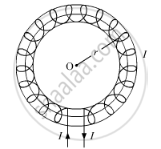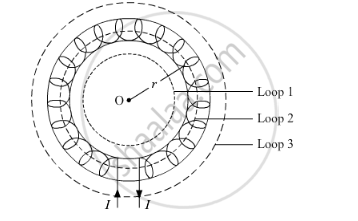# Use this Law to Obtain the Expression for the Magnetic Field Inside an Air Cored Toroid of Average Radius 'r', Having 'n' Turns per Unit Length and Carrying a Steady Current I. - Physics

Use this law to obtain the expression for the magnetic field inside an air cored toroid of average radius 'r', having 'n' turns per unit length and carrying a steady current I.

#### SolutionA toroid is a hollow circular ring on which a large number of turns of a wire are closely wound. Consider an air-cored toroid (as shown above) with centre O.

Given:
r = Average radius of the toroid
I = Current through the solenoid
n = Number of turns per unit length

To determine the magnetic field inside the toroid, we consider three amperian loops (loop 1, loop 2 and loop 3) as show in the figure below.For loop 1:

According to Ampere's circuital law, we have

ointvecB.vec(dl)=mu_0(

Total current for loop 1 is zero because no current is passing through this loop.

So, for loop 1

oint.vecB.vec(dl)=0

For loop 3:

According to Ampere's circuital law, we have

ointvecB.vec(dl)=mu_0(

Total current for loop 3 is zero because net current coming out of this loop is equal to the net current going inside the loop.

For loop 2:

The total current flowing through the toroid is NI, where N is the total number of turns.

ointvecB.vec(dl)=mu_0(NI)

Now, vecB

ointvecB.vec(dl)=Bointdl

=>ointvecB.vec(dl)=B(2pir)

Comparing (i) and (ii), we get

B(2πr)=μ0NI

=>B=(mu_0NI)/(2pir)

Number of turns per unit length is given by

n=N(2pir)

B=μ0nI

This is the expression for magnetic field inside air-cored toroid.

Concept: Solenoid and the Toroid - the Solenoid
Is there an error in this question or solution?